Intermediate Algebra 2e

# 6.3Factor Special Products

Intermediate Algebra 2e6.3 Factor Special Products

### Learning Objectives

By the end of this section, you will be able to:

• Factor perfect square trinomials
• Factor differences of squares
• Factor sums and differences of cubes

### Be Prepared 6.7

Before you get started, take this readiness quiz.

Simplify: $(3x2)3.(3x2)3.$
If you missed this problem, review Example 5.18.

### Be Prepared 6.8

Multiply: $(m+4)2.(m+4)2.$
If you missed this problem, review Example 5.32.

### Be Prepared 6.9

Multiply: $(x−3)(x+3).(x−3)(x+3).$
If you missed this problem, review Example 5.33.

We have seen that some binomials and trinomials result from special products—squaring binomials and multiplying conjugates. If you learn to recognize these kinds of polynomials, you can use the special products patterns to factor them much more quickly.

### Factor Perfect Square Trinomials

Some trinomials are perfect squares. They result from multiplying a binomial times itself. We squared a binomial using the Binomial Squares pattern in a previous chapter.The trinomial $9x2+24x+169x2+24x+16$ is called a perfect square trinomial. It is the square of the binomial $3x+4.3x+4.$

In this chapter, you will start with a perfect square trinomial and factor it into its prime factors.

You could factor this trinomial using the methods described in the last section, since it is of the form $ax2+bx+c.ax2+bx+c.$ But if you recognize that the first and last terms are squares and the trinomial fits the perfect square trinomials pattern, you will save yourself a lot of work.

Here is the pattern—the reverse of the binomial squares pattern.

### Perfect Square Trinomials Pattern

If a and b are real numbers

$a2+2ab+b2=(a+b)2a2−2ab+b2=(a−b)2a2+2ab+b2=(a+b)2a2−2ab+b2=(a−b)2$

To make use of this pattern, you have to recognize that a given trinomial fits it. Check first to see if the leading coefficient is a perfect square, $a2.a2.$ Next check that the last term is a perfect square, $b2.b2.$ Then check the middle term—is it the product, $2ab?2ab?$ If everything checks, you can easily write the factors.

### Example 6.23

#### How to Factor Perfect Square Trinomials

Factor: $9x2+12x+4.9x2+12x+4.$

### Try It 6.45

Factor: $4x2+12x+9.4x2+12x+9.$

### Try It 6.46

Factor: $9y2+24y+16.9y2+24y+16.$

The sign of the middle term determines which pattern we will use. When the middle term is negative, we use the pattern $a2−2ab+b2,a2−2ab+b2,$ which factors to $(a−b)2.(a−b)2.$

The steps are summarized here.

### How To

#### Factor perfect square trinomials.

$Step 1.Does the trinomial fit the pattern?a2+2ab+b2a2−2ab+b2 Is the first term a perfect square?(a)2(a)2 Write it as a square. Is the last term a perfect square?(a)2(b)2(a)2(b)2 Write it as a square. Check the middle term. Is it2ab?(a)2↘2·a·b↙(b)2(a)2↘2·a·b↙(b)2 Step 2.Write the square of the binomial.(a+b)2(a−b)2 Step 3.Check by multiplying.Step 1.Does the trinomial fit the pattern?a2+2ab+b2a2−2ab+b2 Is the first term a perfect square?(a)2(a)2 Write it as a square. Is the last term a perfect square?(a)2(b)2(a)2(b)2 Write it as a square. Check the middle term. Is it2ab?(a)2↘2·a·b↙(b)2(a)2↘2·a·b↙(b)2 Step 2.Write the square of the binomial.(a+b)2(a−b)2 Step 3.Check by multiplying.$

We’ll work one now where the middle term is negative.

### Example 6.24

Factor: $81y2−72y+16.81y2−72y+16.$

### Try It 6.47

Factor: $64y2−80y+25.64y2−80y+25.$

### Try It 6.48

Factor: $16z2−72z+81.16z2−72z+81.$

The next example will be a perfect square trinomial with two variables.

### Example 6.25

Factor: $36x2+84xy+49y2.36x2+84xy+49y2.$

### Try It 6.49

Factor: $49x2+84xy+36y2.49x2+84xy+36y2.$

### Try It 6.50

Factor: $64m2+112mn+49n2.64m2+112mn+49n2.$

Remember the first step in factoring is to look for a greatest common factor. Perfect square trinomials may have a GCF in all three terms and it should be factored out first. And, sometimes, once the GCF has been factored, you will recognize a perfect square trinomial.

### Example 6.26

Factor: $100x2y−80xy+16y.100x2y−80xy+16y.$

### Try It 6.51

Factor: $8x2y−24xy+18y.8x2y−24xy+18y.$

### Try It 6.52

Factor: $27p2q+90pq+75q.27p2q+90pq+75q.$

### Factor Differences of Squares

The other special product you saw in the previous chapter was the Product of Conjugates pattern. You used this to multiply two binomials that were conjugates. Here’s an example: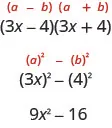A difference of squares factors to a product of conjugates.

### Difference of Squares Pattern

If a and b are real numbers,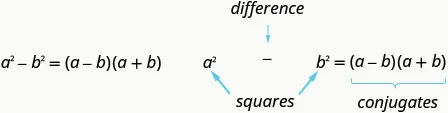Remember, “difference” refers to subtraction. So, to use this pattern you must make sure you have a binomial in which two squares are being subtracted.

### Example 6.27

#### How to Factor a Binomial Using the Difference of Squares

Factor: $64y2−1.64y2−1.$

### Try It 6.53

Factor: $121m2−1.121m2−1.$

### Try It 6.54

Factor: $81y2−1.81y2−1.$

### How To

#### Factor differences of squares.

$Step 1.Does the binomial fit the pattern?a2−b2Is this a difference?____−____Are the first and last terms perfect squares?Step 2.Write them as squares.(a)2−(b)2Step 3.Write the product of conjugates.(a−b)(a+b)Step 4.Check by multiplying.Step 1.Does the binomial fit the pattern?a2−b2Is this a difference?____−____Are the first and last terms perfect squares?Step 2.Write them as squares.(a)2−(b)2Step 3.Write the product of conjugates.(a−b)(a+b)Step 4.Check by multiplying.$

It is important to remember that sums of squares do not factor into a product of binomials. There are no binomial factors that multiply together to get a sum of squares. After removing any GCF, the expression $a2+b2a2+b2$ is prime!

The next example shows variables in both terms.

### Example 6.28

Factor: $144x2−49y2.144x2−49y2.$

### Try It 6.55

Factor: $196m2−25n2.196m2−25n2.$

### Try It 6.56

Factor: $121p2−9q2.121p2−9q2.$

As always, you should look for a common factor first whenever you have an expression to factor. Sometimes a common factor may “disguise” the difference of squares and you won’t recognize the perfect squares until you factor the GCF.

Also, to completely factor the binomial in the next example, we’ll factor a difference of squares twice!

### Example 6.29

Factor: $48x4y2−243y2.48x4y2−243y2.$

### Try It 6.57

Factor: $2x4y2−32y2.2x4y2−32y2.$

### Try It 6.58

Factor: $7a4c2−7b4c2.7a4c2−7b4c2.$

The next example has a polynomial with 4 terms. So far, when this occurred we grouped the terms in twos and factored from there. Here we will notice that the first three terms form a perfect square trinomial.

### Example 6.30

Factor: $x2−6x+9−y2.x2−6x+9−y2.$

### Try It 6.59

Factor: $x2−10x+25−y2.x2−10x+25−y2.$

### Try It 6.60

Factor: $x2+6x+9−4y2.x2+6x+9−4y2.$

### Factor Sums and Differences of Cubes

There is another special pattern for factoring, one that we did not use when we multiplied polynomials. This is the pattern for the sum and difference of cubes. We will write these formulas first and then check them by multiplication.

$a3+b3=(a+b)(a2−ab+b2)a3−b3=(a−b)(a2+ab+b2)a3+b3=(a+b)(a2−ab+b2)a3−b3=(a−b)(a2+ab+b2)$

We’ll check the first pattern and leave the second to you.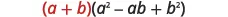Distribute.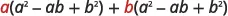Multiply.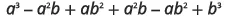Combine like terms.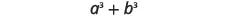### Sum and Difference of Cubes Pattern

$a3+b3=(a+b)(a2−ab+b2)a3−b3=(a−b)(a2+ab+b2)a3+b3=(a+b)(a2−ab+b2)a3−b3=(a−b)(a2+ab+b2)$

The two patterns look very similar, don’t they? But notice the signs in the factors. The sign of the binomial factor matches the sign in the original binomial. And the sign of the middle term of the trinomial factor is the opposite of the sign in the original binomial. If you recognize the pattern of the signs, it may help you memorize the patterns.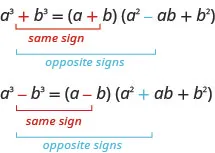The trinomial factor in the sum and difference of cubes pattern cannot be factored.

It will be very helpful if you learn to recognize the cubes of the integers from 1 to 10, just like you have learned to recognize squares. We have listed the cubes of the integers from 1 to 10 in Table 6.1.

n 1 2 3 4 5 6 7 8 9 10
$n3n3$ 1 8 27 64 125 216 343 512 729 1000
Table 6.1

### Example 6.31

#### How to Factor the Sum or Difference of Cubes

Factor: $x3+64.x3+64.$

### Try It 6.61

Factor: $x3+27.x3+27.$

### Try It 6.62

Factor: $y3+8.y3+8.$

### How To

#### Factor the sum or difference of cubes.

1. Step 1. Does the binomial fit the sum or difference of cubes pattern?
Is it a sum or difference?
Are the first and last terms perfect cubes?
2. Step 2. Write them as cubes.
3. Step 3. Use either the sum or difference of cubes pattern.
4. Step 4. Simplify inside the parentheses.
5. Step 5. Check by multiplying the factors.

### Example 6.32

Factor: $27u3−125v3.27u3−125v3.$

### Try It 6.63

Factor: $8x3−27y3.8x3−27y3.$

### Try It 6.64

Factor: $1000m3−125n3.1000m3−125n3.$

In the next example, we first factor out the GCF. Then we can recognize the sum of cubes.

### Example 6.33

Factor: $6x3y+48y4.6x3y+48y4.$

### Try It 6.65

Factor: $500p3+4q3.500p3+4q3.$

### Try It 6.66

Factor: $432c3+686d3.432c3+686d3.$

The first term in the next example is a binomial cubed.

### Example 6.34

Factor: $(x+5)3−64x3.(x+5)3−64x3.$

### Try It 6.67

Factor: $(y+1)3−27y3.(y+1)3−27y3.$

### Try It 6.68

Factor: $(n+3)3−125n3.(n+3)3−125n3.$

### Media

Access this online resource for additional instruction and practice with factoring special products.

### Section 6.3 Exercises

#### Practice Makes Perfect

Factor Perfect Square Trinomials

In the following exercises, factor completely using the perfect square trinomials pattern.

159.

$16 y 2 + 24 y + 9 16 y 2 + 24 y + 9$

160.

$25 v 2 + 20 v + 4 25 v 2 + 20 v + 4$

161.

$36 s 2 + 84 s + 49 36 s 2 + 84 s + 49$

162.

$49 s 2 + 154 s + 121 49 s 2 + 154 s + 121$

163.

$100 x 2 − 20 x + 1 100 x 2 − 20 x + 1$

164.

$64 z 2 − 16 z + 1 64 z 2 − 16 z + 1$

165.

$25 n 2 − 120 n + 144 25 n 2 − 120 n + 144$

166.

$4 p 2 − 52 p + 169 4 p 2 − 52 p + 169$

167.

$49 x 2 + 28 x y + 4 y 2 49 x 2 + 28 x y + 4 y 2$

168.

$25 r 2 + 60 r s + 36 s 2 25 r 2 + 60 r s + 36 s 2$

169.

$100 y 2 − 20 y + 1 100 y 2 − 20 y + 1$

170.

$64 m 2 − 16 m + 1 64 m 2 − 16 m + 1$

171.

$10 j k 2 + 80 j k + 160 j 10 j k 2 + 80 j k + 160 j$

172.

$64 x 2 y − 96 x y + 36 y 64 x 2 y − 96 x y + 36 y$

173.

$75 u 4 − 30 u 3 v + 3 u 2 v 2 75 u 4 − 30 u 3 v + 3 u 2 v 2$

174.

$90 p 4 + 300 p 3 q + 250 p 2 q 2 90 p 4 + 300 p 3 q + 250 p 2 q 2$

Factor Differences of Squares

In the following exercises, factor completely using the difference of squares pattern, if possible.

175.

$25 v 2 − 1 25 v 2 − 1$

176.

$169 q 2 − 1 169 q 2 − 1$

177.

$4 − 49 x 2 4 − 49 x 2$

178.

$121 − 25 s 2 121 − 25 s 2$

179.

$6 p 2 q 2 − 54 p 2 6 p 2 q 2 − 54 p 2$

180.

$98 r 3 − 72 r 98 r 3 − 72 r$

181.

$24 p 2 + 54 24 p 2 + 54$

182.

$20 b 2 + 140 20 b 2 + 140$

183.

$121 x 2 − 144 y 2 121 x 2 − 144 y 2$

184.

$49 x 2 − 81 y 2 49 x 2 − 81 y 2$

185.

$169 c 2 − 36 d 2 169 c 2 − 36 d 2$

186.

$36 p 2 − 49 q 2 36 p 2 − 49 q 2$

187.

$16 z 4 − 1 16 z 4 − 1$

188.

$m 4 − n 4 m 4 − n 4$

189.

$162 a 4 b 2 − 32 b 2 162 a 4 b 2 − 32 b 2$

190.

$48 m 4 n 2 − 243 n 2 48 m 4 n 2 − 243 n 2$

191.

$x 2 − 16 x + 64 − y 2 x 2 − 16 x + 64 − y 2$

192.

$p 2 + 14 p + 49 − q 2 p 2 + 14 p + 49 − q 2$

193.

$a 2 + 6 a + 9 − 9 b 2 a 2 + 6 a + 9 − 9 b 2$

194.

$m 2 − 6 m + 9 − 16 n 2 m 2 − 6 m + 9 − 16 n 2$

Factor Sums and Differences of Cubes

In the following exercises, factor completely using the sums and differences of cubes pattern, if possible.

195.

$x 3 + 125 x 3 + 125$

196.

$n 6 + 512 n 6 + 512$

197.

$z 6 − 27 z 6 − 27$

198.

$v 3 − 216 v 3 − 216$

199.

$8 − 343 t 3 8 − 343 t 3$

200.

$125 − 27 w 3 125 − 27 w 3$

201.

$8 y 3 − 125 z 3 8 y 3 − 125 z 3$

202.

$27 x 3 − 64 y 3 27 x 3 − 64 y 3$

203.

$216 a 3 + 125 b 3 216 a 3 + 125 b 3$

204.

$27 y 3 + 8 z 3 27 y 3 + 8 z 3$

205.

$7 k 3 + 56 7 k 3 + 56$

206.

$6 x 3 − 48 y 3 6 x 3 − 48 y 3$

207.

$2 x 2 − 16 x 2 y 3 2 x 2 − 16 x 2 y 3$

208.

$−2 x 3 y 2 − 16 y 5 −2 x 3 y 2 − 16 y 5$

209.

$( x + 3 ) 3 + 8 x 3 ( x + 3 ) 3 + 8 x 3$

210.

$( x + 4 ) 3 − 27 x 3 ( x + 4 ) 3 − 27 x 3$

211.

$( y − 5 ) 3 − 64 y 3 ( y − 5 ) 3 − 64 y 3$

212.

$( y − 5 ) 3 + 125 y 3 ( y − 5 ) 3 + 125 y 3$

Mixed Practice

In the following exercises, factor completely.

213.

$64 a 2 − 25 64 a 2 − 25$

214.

$121 x 2 − 144 121 x 2 − 144$

215.

$27 q 2 − 3 27 q 2 − 3$

216.

$4 p 2 − 100 4 p 2 − 100$

217.

$16 x 2 − 72 x + 81 16 x 2 − 72 x + 81$

218.

$36 y 2 + 12 y + 1 36 y 2 + 12 y + 1$

219.

$8 p 2 + 2 8 p 2 + 2$

220.

$81 x 2 + 169 81 x 2 + 169$

221.

$125 − 8 y 3 125 − 8 y 3$

222.

$27 u 3 + 1000 27 u 3 + 1000$

223.

$45 n 2 + 60 n + 20 45 n 2 + 60 n + 20$

224.

$48 q 3 − 24 q 2 + 3 q 48 q 3 − 24 q 2 + 3 q$

225.

$x 2 − 10 x + 25 − y 2 x 2 − 10 x + 25 − y 2$

226.

$x 2 + 12 x + 36 − y 2 x 2 + 12 x + 36 − y 2$

227.

$( x + 1 ) 3 + 8 x 3 ( x + 1 ) 3 + 8 x 3$

228.

$( y − 3 ) 3 − 64 y 3 ( y − 3 ) 3 − 64 y 3$

#### Writing Exercises

229.

Why was it important to practice using the binomial squares pattern in the chapter on multiplying polynomials?

230.

How do you recognize the binomial squares pattern?

231.

Explain why $n2+25≠(n+5)2.n2+25≠(n+5)2.$ Use algebra, words, or pictures.

232.

Maribel factored $y2−30y+81y2−30y+81$ as $(y−9)2.(y−9)2.$ Was she right or wrong? How do you know?

#### Self Check

After completing the exercises, use this checklist to evaluate your mastery of the objectives of this section.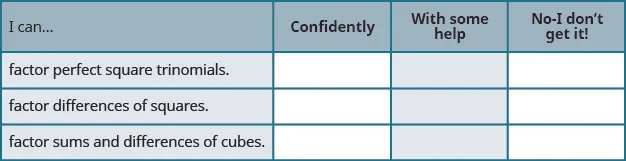What does this checklist tell you about your mastery of this section? What steps will you take to improve?

Order a print copy

As an Amazon Associate we earn from qualifying purchases.